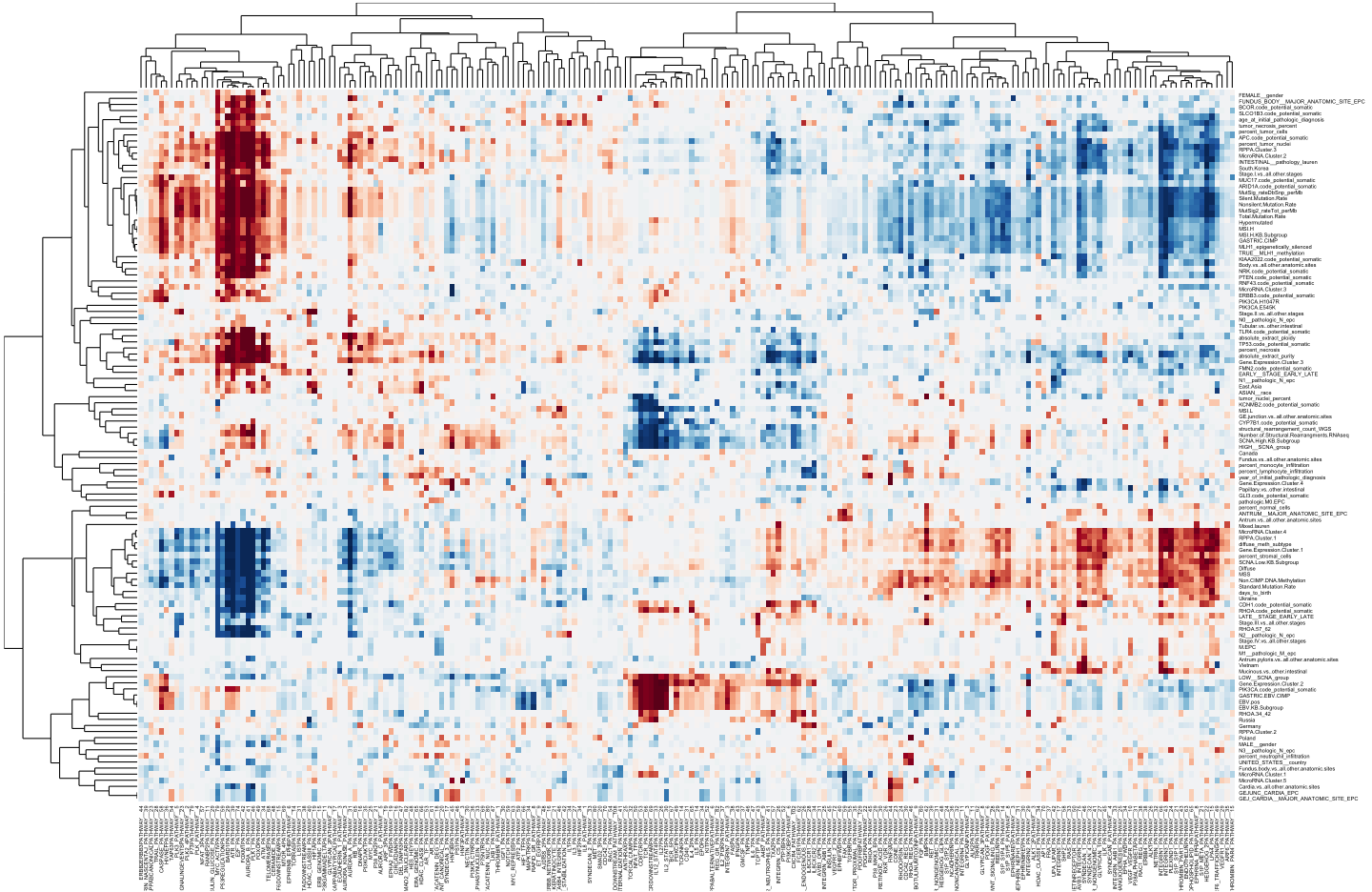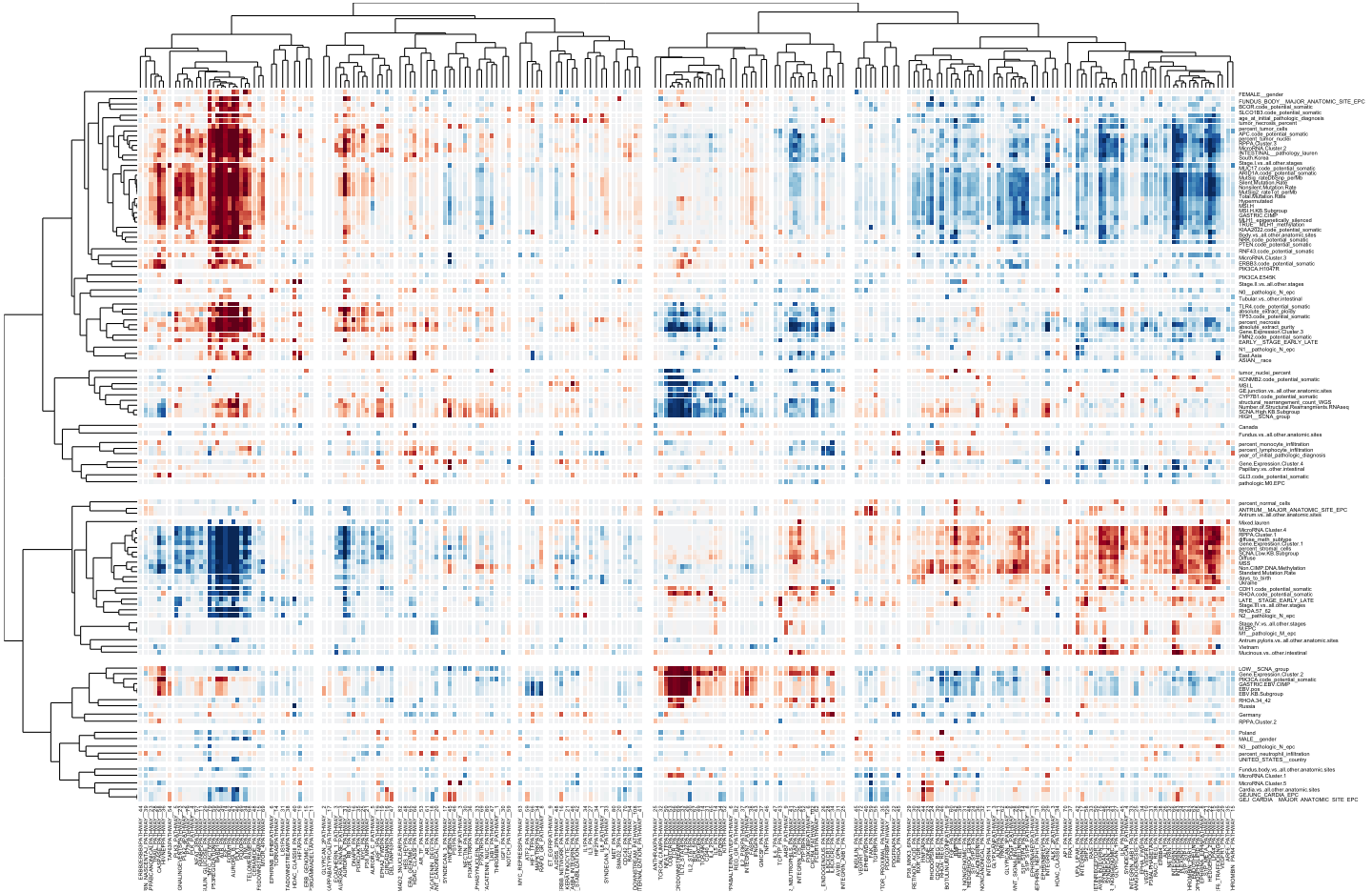title: “tcga_example” author: “Ryo Sakai” date: “8 September 2014”

## output: html_document

This is an R Markdown document, which demonstrates the use of gapmap and dendsort packages to generate a gapped cluster heatmap visualization.

Let's start by loading the data file from the package, and creating two color palettes.

library(gapmap)
data("sample_tcga")
library(RColorBrewer)
RdBu = rev(brewer.pal(11, name="RdBu"))
RdYlBu = rev(brewer.pal(11, name="RdYlBu"))


Now you have the data matrix loaded, let's calculate correlation-based distance and perform hierarchical clustering. In this example, we use the correlation-based dissimilarity (Pearson Correlation) and the complete linkage for hierarchical clustering.

#transpose
dataTable <- t(sample_tcga)
#calculate the correlation based distance
row_dist <- as.dist(1-cor(t(dataTable), method = "pearson"))
col_dist <- as.dist(1-cor(dataTable, method = "pearson"))
#hierarchical clustering
col_hc <- hclust(col_dist, method = "complete")
row_hc <- hclust(row_dist, method = "complete")
col_d <- as.dendrogram(col_hc)
row_d <- as.dendrogram(row_hc)


Now you are ready to plot the data. First, we will plot a cluster heatmap without any gaps.

gapmap(m = as.matrix(dataTable), d_row = rev(row_d), d_col = col_d, ratio = 0, verbose=FALSE, col=RdBu,
label_size=2, v_ratio= c(0.1,0.8,0.1), h_ratio=c(0.1,0.8,0.1))This gapmap package was designed to encode the similarity between two nodes by adjusting the position of each leaves. In the traditional representation, all the information about the similarity two adjacent nodes is in the height of the branch in a dendrogram. By positioning leaves based on the similarity, we introduce gaps in both dendrograms and heat map visualization. In the figure below, we exponentially map a distance (dissimilarity) of two nodes to a scale of gap size.

gapmap(m = as.matrix(dataTable), d_row = rev(row_d), d_col = col_d, mode = "quantitative", mapping="exponential", col=RdBu,
ratio = 0.3, verbose=FALSE, scale = 0.5, label_size=2, v_ratio= c(0.1,0.8,0.1), h_ratio=c(0.1,0.8,0.1))Since the background is white, we can use another color scale where the value 0 is encoded in yellow.

gapmap(m = as.matrix(dataTable), d_row = rev(row_d), d_col = col_d, mode = "quantitative", mapping="exponential", col=RdYlBu,
ratio = 0.3, verbose=FALSE, scale = 0.5, label_size=2, v_ratio= c(0.1,0.8,0.1), h_ratio=c(0.1,0.8,0.1))